# Planimetrics - math word problems

Study plane measurements, including angles, distances, and areas. In other words - measurement and calculation of shapes in the plane. Perimeter and area of plane shapes.

#### Number of problems found: 1949

• Paper squaresThe paper rectangle measuring 69 cm and 46 cm should be cut into as many squares as possible. Calculate the lengths of squares and their number.
• Two squaresTwo squares whose sides are in the ratio 5:2 have a sum of its perimeters 73 cm. Calculate the sum of the area of these two squares.
• Common chordTwo circles with radius 17 cm and 20 cm are intersect at two points. Its common chord is long 27 cm. What is the distance of the centers of these circles?
• Round flowerbed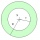Around a round flowerbed with a diameter of 6 meters and I will make a sidewalk up to 0.5 meters wide. How much gravel is needed if the layer is to be 5 cm high?
• Negative and postcard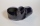The image on the negative has dimensions of 36x24 mm and is enlarged to a postcard format of 13.5x9 cm. In what proportion have the lengths increased? In what proportion has the image content increased?The regular quadrangular pyramid has a base length of 6 cm and a side edge length of 9 centimeters. Calculate its volume and surface area.
• Chimney and treeCalculate the height of the factory chimney, which casts a shadow 6.5 m long in the afternoon. At the same time, a 6 m high tree standing near it casts a shadow 25 dm long.
• IcebergWhat is the surface area of 50 cm iceberg (in the shape of a cuboid) that can carry a man with luggage with a total weight of 120 kg?
• Two 2D shapes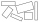Decide which shapes have more area:   (a) a square of 8cm side; or   (b) two rectangles with sides 5cm and 15cm? Write result as 1 or 2 (rectangles)
• 30-60-90The longer leg of a 30°-60°-90° triangle measures 5. What is the length of the shorter leg?
• SeedsThe field has a rectangular shape with dimensions of 128 m and 350 m. How many kg of seed are needed for sowing if the 1 m2 will consume 25 g of seeds?
• Train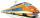The train is running at speeds of 96 km/h. From the beginning of braking to full stop train run for 3.3 minutes. If the train slows the braking equally, calculate the distance of the place from the station where you need to start to brake.
• Brass sphereFind the weight of a brass ball with an outer radius of 12 cm, a wall thickness of 20 mm if the density of the brass is 8.5 g/cm3.
• RTriangle 17The hypotenuse of a right triangle is 17 cm. If you decrease both two legs by 3 cm you will reduce the hypotenuse by 4 cm. Determine the length of this legs.
• The bus stop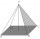The bus stop waiting room has the shape of a regular quadrilateral pyramid 4 m high with a 5 m base edge. Calculate how many m2 roofing is required to cover the three walls of the sheathing, taking into account 40% of the additional coverage.
• Arc and segmentCalculate the length of circular arc l, area of the circular arc S1 and area of circular segment S2. Radius of the circle is 11 and corresponding angle is ?.
• Flower bed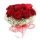The park has a rectangular flower bed with dimensions of 3.2m and 1.5m. How many rose bushes will we plant in the flower bed if 20 dm2 are needed for one bush?
• Medians in right triangleIt is given a right triangle, angle C is 90 degrees. I know it medians t1 = 8 cm and median t2 = 12 cm. .. How to calculate the length of the sides?
• Square prismCalculate the volume of a foursided prism 2 dm high, the base is a trapezoid with bases 12 cm, 6 cm, height of 4 cm and 5 cm long arms.
• Cutting paperDivide a rectangular paper with dimensions 220mm and 308mm into squares of the same size so that they are as large as possible. Specify the length of the side of the square.

Do you have an interesting mathematical word problem that you can't solve it? Submit a math problem, and we can try to solve it.

We will send a solution to your e-mail address. Solved examples are also published here. Please enter the e-mail correctly and check whether you don't have a full mailbox.

Please do not submit problems from current active competitions such as Mathematical Olympiad, correspondence seminars etc...